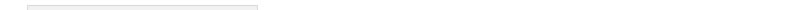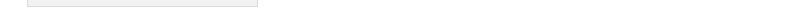Sitemap Print Version Mailform Login Last update:May 06. 2011 11:40:11

Homework assignments
Matlab files
• sqgrid.m - Generates a mesh on a square
• lapdir.m - 5-point matrix for the Dirichlet problem for the Poisson equation
• square.m - An example driver file that uses the preceding two functions
• bump.m - Tent function to be used as an initial condition
• advection.m - First order finite difference solver for the advection equation
• widebump.m - Wider tent function to be used as an initial condition
• widebump1.m - Tilted tent function to be used as an initial condition
• heat.m - Explicit finite difference solver for the heat equation
• heatimp.m - Implicit finite difference solver for the heat equation
• smoothbump.m - Smoother bump function suitable for wave.m
• wave.m - Finite difference solver for the wave equation
Mathematica files
Lecture slidesMATH 319: Introduction to Partial Differential Equations Winter 2011